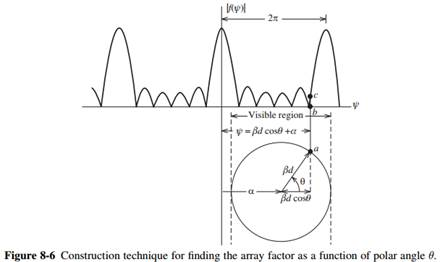### Create an Account

Home / Questions / Use the techniques of Fig 86 to obtain a polar plot of the array factor of the array given in

# Use the techniques of Fig 86 to obtain a polar plot of the array factor of the array given in

Use the techniques of Fig. 8-6 to obtain a polar plot of the array factor of the array given in Prob. 8.2-1.Problem 8.2-1

Consider an array of two elements spaced one wavelength apart with currents that are equal in amplitude and 180° out-of-phase.

(a) Use the inspection method to rough sketch the polar plot of the array factor.

(b) Derive the exact array factor as a function of θ if the elements are on the z-axis.

(c) For what angles of θ is this array factor maximum?

(d) What is the expression for the normalized array factor |f(θ)|?Subscribe To Get Solution